# Preface

This section presents material on complex Fourier series including various examples.

Introduction to Linear Algebra with Mathematica

# Complex Fourier Series

The complex exponential form of Fourier series is a representation of a periodic function (which is usually a signal) with period $$2\ell$$ as infinite series:
\begin{equation} \label{EqComplex.1} f(x) \,\sim\, \mbox{P.V.} \sum_{k=-\infty}^{\infty} \alpha_k e^{k{\bf j} \pi x/\ell} \qquad ({\bf j}^2 = -1), \end{equation}
where a signal's complex Fourier spectrum is
\begin{equation} \label{EqComplex.2} \alpha_k = \frac{1}{2\ell} \int_{-\ell}^{\ell} f(x)\, e^{-k{\bf j} \pi x/\ell} \,{\text d} x , \qquad k=0, \pm 1, \pm 2, \ldots ; \end{equation}
provided that this series converges in some sense. The abbreviation P.V. means the Cauchy principal value regularization:
\begin{equation} \label{EqComplex.3} \mbox{P.V.} \sum_{k=-\infty}^{\infty} \alpha_k e^{k{\bf j} \pi x/\ell} = \lim_{N\to \infty} \sum_{k=-N}^{N} \alpha_k e^{k{\bf j} \pi x/\ell} \end{equation}
because restoring a function from its Fourier coefficients is an ill-posed problem. Formula \eqref{EqComplex.2} is based on the orthogonality property of exponential functions:
$\int_0^T e^{{\bf j}2\pi kt/T} \,e^{-{\bf j}2\pi nt/T} \,{\text d}t = \left\{ \begin{array}{ll} T, & \ \mbox{if k=n}, \\ 0 , & \ \mbox{if } k\ne n . \end{array} \right.$
The conversion of complex Fourier series into standard trigonometric Fourier series is based on Euler's formulas:

$\sin \theta = \frac{1}{2{\bf j}} \,e^{{\bf j}\theta} - \frac{1}{2{\bf j}} \,e^{-{\bf j}\theta} = \Im \,e^{{\bf j}\theta} = \mbox{Im} \,e^{{\bf j}\theta}, \qquad \cos \theta = \frac{1}{2} \,e^{{\bf j}\theta} - \frac{1}{2} \,e^{-{\bf j}\theta} = \Re \,e^{{\bf j}\theta} = \mbox{Re} \,e^{{\bf j}\theta}.$

Here, j is the unit vector in positive vertical direction on the complex plane, so $${\bf j}^2 =-1.$$ For example the Fourier series for the Dirac delta function on a symmetric interval (−&ell, ℓ) is

\begin{equation} \label{EqComplex.4} \delta (x) = \mbox{P.V.} \frac{1}{2\ell} \sum_{k=-\infty}^{\infty} e^{-k{\bf j} \pi x/\ell} =\frac{1}{2\ell} + \frac{1}{\ell}\,\lim_{N\to \infty} \sum_{k=1}^N \cos \left( \frac{k\pi x}{\ell} \right) = \frac{1}{2\ell}\,\lim_{N\to \infty} \frac{\sin \left( 2N+1 \right) \frac{x\pi}{2\ell}}{\sin \frac{x\pi}{2\ell}} . \end{equation}
This means that for every probe function f(x) on the inverval −ℓ < x < ℓ,
$\frac{1}{2\ell}\,\lim_{N\to \infty} \int_{-\ell}^{\ell} {\text d}x\,f(x)\,\frac{\sin \left( 2N+1 \right) \frac{x\pi}{2\ell}}{\sin \frac{x\pi}{2\ell}} = f(0) .$

Mathematica has a default command to calculate complex Fourier series:

FourierSeries[ expr, t, n]        (* gives the n-order (complex) Fourier series expansion of expr in t *)

Mathematica has a special command to find complex Fourier coefficient and to determine its numerical approximation:

FourierCoefficient[ expr, t, n]        (* gives the nth coefficient in the exponential Fourier series expansion of expr in t *)

NFourierCoefficient[ expr, t, n]        (* gives a numerical approximation to the nth coefficient in the Fourier exponential series expansion of expr in t *)

One can easily transfer complex form into trigonometric form vice versa using formulas:

$\alpha_k = \begin{cases} \frac{a_0}{2} , & \quad k=0, \\ \frac{1}{2} \left( a_k - {\bf j} b_k \right) , & \quad k=1,2,3,\ldots , \\ \frac{1}{2} \left( a_{-k} + {\bf j} b_{-k} \right) , & \quad k=-1,-2,-3,\ldots \end{cases}$
and
$a_0 = 2\,\alpha_0 , \quad a_k = 2\,\Re \alpha_k = 2\,\mbox{Re} \,\alpha_k , \quad b_k = -2\,\Im \alpha_k = -2\,\mbox{Im} \,\alpha_k , \quad k=1,2,\ldots .$
Note that $$a_{-n} \quad\mbox{and}\quad b_{-n}$$ are only defined when n is negative. Then we get
$f(x) \,\sim\, \sum_{k=-\infty}^{\infty} \alpha_k e^{k{\bf j} \pi x/\ell} = \frac{a_0}{2} + \sum_{n\ge 1} \left[ a_n \cos \frac{n\pi x}{\ell} + b_n \sin \frac{n\pi x}{\ell} \right] .$

The Fourier series command has an option FourierParameters that involves two parameters and when applied, it looks as FourierParameters->{a,b}
This means that complex Fourier coefficient is evaluated according to the formula:

$\left\vert \frac{b}{2\,\pi} \right\vert^{(a+1)/2} \, \int_{-\pi/|b|}^{\pi/|b|} f(t)\,e^{-{\bf j}bnt} \,{\text d}t .$

Example 1: Consider a piecewise constant function on the interval [-2 , 2]:

$f(x) = \left\{ \begin{array}{ll} 1, & \ \mbox{on the interval } -2 < x < -1, \\ 0 , & \ \mbox{on the interval } -1 < x < 0 , \\ 2, & \ \mbox{on the interval } 0 < x < 2. \end{array} \right.$
There is no need to define the function at the points of discontinuity $$x=-2, -1, 0, 2$$ because the corresponding Fourier series will specify the values at these points to be the averages of left and right limit values. Therefore, $$f(-2) = 3/2, \ f(-1) = 1/2, \ f(0) = 1, \ f(2) =3/2 .$$ We can find the Fourier coefficients either by evaluating integrals
$\alpha_0 = \frac{1}{4} \,\int_{-2}^2 f(x)\,{\text d}x = \frac{5}{4} , \qquad \alpha_k = \frac{1}{4} \,\int_{-2}^2 f(x)\,e^{-k{\bf j} \pi x/2} \,{\text d}x = \frac{\bf j}{2k\pi} \left[ (-1)^k + \cos \frac{k\pi}{2} -2 \right] - \frac{1}{2k\pi} \,\sin \frac{k\pi}{2} , \quad k = \pm 1, \pm 2, \ldots .$
f[t_] = Piecewise[{{1, -2 < t < -1}, {0, -1 < t < 0}, {2, 0 < t < 2}}]
Integrate[f[t]*Exp[-k*I*Pi*t/2], {t, -2, 2}]/4 // ComplexExpand
Simplify[%]
Out= (I (Cos[(k $Pi])/2] + Cos[k \[Pi]] + I (2 I + Sin[(k \[Pi])/2] - 3 Sin[k \[Pi]])))/(2 k \[Pi]) or using standard command: FourierSeries[f[x], x, 5, FourierParameters -> {1, Pi/2}] // ComplexExpand 5/4 - Cos[(\[Pi] x)/2]/\[Pi] + Cos[(3 \[Pi] x)/2]/(3 \[Pi]) - Cos[(5 \[Pi] x)/2]/(5 \[Pi]) + (3 Sin[(\[Pi] x)/2])/\[Pi] + Sin[\[Pi] x]/\[Pi] + Sin[(3 \[Pi] x)/2]/\[Pi] + ( 3 Sin[(5 \[Pi] x)/2])/(5 \[Pi]) The corresponding real trigonometric series is \[ f(t) = \frac{5}{4} - \frac{1}{\pi} \,\sum_{k\ge 1} \frac{1}{k}\,\sin \frac{k\pi}{2}\, \cos \frac{k\pi t}{2} - \frac{1}{\pi} \,\sum_{k\ge 1} \frac{1}{k}\left[ \cos \frac{k\pi}{2} + (-1)^k -2 \right] \sin \frac{k\pi t}{2} .$
You can find a single Fourier coefficient (only for complex form) with a command
FourierCoefficient[f[t], t, 5, FourierParameters -> {1, Pi/2}]
-((1/10 + (3 I)/10)/$Pi]) and when you add to FourierCoefficient[f[t], t, -5, FourierParameters -> {1, Pi/2}] -((1/10 - (3 I)/10)/\[Pi]) it will provide you with a simplified answer. Correctness of calculations could be checked numerically: NFourierCoefficient[f[t], t, 5, FourierParameters -> {1, Pi/2}] Out= -0.031831 - 0.095493 I NFourierCoefficient[f[t], t, -5, FourierParameters -> {1, Pi/2}] Out= -0.031831 + 0.095493 I N[Sin[5*Pi/2]/10/Pi] Out= 0.031831 N[3/10/Pi] Out= 0.095493 because $$(-1)^5 + \cos \frac{5\pi}{2} -2 = -3 .$$ To compare the quality of Fourier approximations, we plot partial sums with 10 and 50 terms, respectively: curve = 5/4 - (1/Pi)* Sum[Sin[k*Pi/2]*Cos[k*Pi*x/2]/k, {k, 1, 10}] - (1/Pi)* Sum[(Cos[k*Pi/2] + (-1)^k - 2)*Sin[k*Pi*x/2]/k, {k, 1, 10}] Plot[curve, {x, -3.5, 3.5}, PlotStyle -> Thick]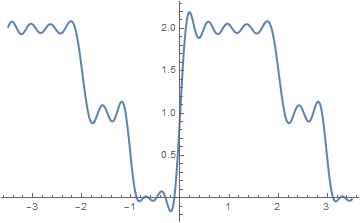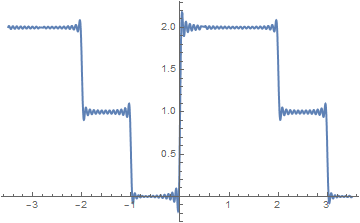When the complex Fourier series is used to represent a periodic function, then the amplitude spectrum, sketched below, is two-sided. It consists of the points $$\left( \frac{k\pi}{\ell} , \left\vert \alpha_k \right\vert \right) , \quad k= 0, \pm 1, \pm 2, \ldots$$ that in our case become \[ \left( 0, \frac{5}{4} \right) , \quad \left( \frac{k\pi}{2}, \frac{1}{2k\pi} \,\sqrt{\left( (-1)^k + \cos \frac{k\pi}{2} -2 \right)^2 + \sin^2 \frac{k\pi}{2}} \right) , \quad k=\pm 1, \pm 2, \ldots .$
a[k_] = (-1)^k + Cos[k*Pi/2] - 2
b[k_] = Sin[k*Pi/2]
p0 = Line[{{0, 0}, {0, 5/4}}]; q0 = Graphics[{Thick, p0}]
pm1 = Line[{{-1*Pi/2, 0}, {-1*Pi/2, Sqrt[(a[-1])^2 + (b[-1])^2]/2/Pi}}]
qm1 = Graphics[{Thick, pm1}]
p1 = Line[{{1*Pi/2, 0}, {1*Pi/2, Sqrt[(a)^2 + (b)^2]/2/Pi}}]
q1 = Graphics[{Thick, p1}]
a = Graphics[Arrow[{{-3*N[Pi]/1.9, 0}, {3*N[Pi]/1.7, 0}}]]
Show[a, q0, q1, q2, q3, qm1, qm2, qm3]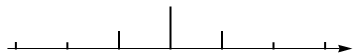The two-sided amplitude spectrum of the function.

The power spectrum for f is also two-sided, consisting of the points $$\left( \frac{k\pi}{\ell} , \left\vert \alpha_k \right\vert^2 \right) , \quad k= 0, \pm 1, \pm 2, \ldots$$ that in our case become
$\left( 0, \frac{5}{4} \right) , \quad \left( \frac{k\pi}{2}, \frac{1}{4k^2\pi^2} \,\left[ \left( (-1)^k + \cos \frac{k\pi}{2} -2 \right)^2 + \sin^2 \frac{k\pi}{2} \right] \right) , \quad k=\pm 1, \pm 2, \ldots .$
f[t_] = Piecewise[{{1, -2 < t < -1}, {0, -1 < t < 0}, {2, 0 < t < 2}}]
DiscretePlot[ Abs[FourierCoefficient[f[x], x, k, FourierParameters -> {1, Pi/2}]]^2, {k, -7, 7}, PlotRange -> All, PlotStyle -> {Thick, PointSize[0.03]}]
Note that here we used Mathematica's command FourierCoefficient that gives the kth coefficient in the Fourier series expansion of f.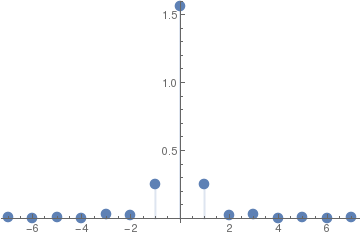The two-sided power spectrum of the function.

■

The complex exponential Fourier form has the following advantages compared to the traditional trigonometric form:

• only need to perform one integration;
• a single exponential can be manipulated more easily than a sum of sinusoidal terms, and
• it provides a logical transition into a further discussion of the Fourier Transform.
A signal's Fourier series spectrum $$\alpha_k$$ has interesting properties.
• If f(t) is a real function, then $$\alpha_{-k} = \overline{\alpha_k} ;$$
• if f(t) is an even function, then $$\alpha_{-k} = \alpha_k ;$$ if f(t) is an odd function, then $$\alpha_{-k} = -\alpha_k ,$$ , and
• the Fourier series obeys Parseval's Theorem: $$\int_0^T f^2 (t) \,{\text d}t = T\,\sum_k \left\vert \alpha_k \right\vert^2 .$$
The following formula may be helpful for evaluating Fourier coefficients:
\begin{align*} \int p(x)\, e^{ax}\,{\text d}x &= \frac{1}{a} \, e^{ax}\,\sum_{k=0}^n \frac{(-1)^k}{a^k} \,{\texttt D}_x^k p(x) \qquad\quad \left( {\texttt D}_x = \frac{\text d}{{\text d}x} \right) \\ &= \frac{1}{a} \, e^{ax} \left[ p(x) - \frac{p' (x)}{a} + \frac{p'' (x)}{a^2} - \cdots + (-1)^n \,\frac{p^{(n)} (x)}{a^n} \right] , \end{align*}
where $$p(x) = b_n x^n + b_{n-1} x^{n-1} + \cdots + b_1 x + b_0$$ is a polynomial of degree n.

Example 2: Find complex and regular Fourier series expansion of the function $$f(x) = \frac{1-a\,\cos x}{1- 2a\,\cos x + a^2} ,$$ where real number a has absolute value less than 1: $$|a| <1 .$$

First, we substitute Euler's representation $$\cos x = \frac{1}{2}\,e^{{\bf j}x} + \frac{1}{2}\,e^{-{\bf j}x} .$$ instead of cos(x). Then

$f(x) = \frac{1 - \frac{a}{2} \left( e^{{\bf j}x} + e^{-{\bf j}x} \right)}{1 - a \left( e^{{\bf j}x} + e^{-{\bf j}x} \right) + a^2} = \frac{1}{2}\,\frac{2- a\, e^{{\bf j}x} - a\,e^{-{\bf j}x}}{\left( 1-a\,e^{{\bf j}x} \right) \left( 1-a\,e^{-{\bf j}x} \right)} .$
Since the numerator can be written as
$2- a\, e^{{\bf j}x} - a\,e^{-{\bf j}x} = 1- a\, e^{{\bf j}x} + 1 - a\, e^{-{\bf j}x} ,$
we simplify the given function as
$f(x) = \frac{1}{2}\,\frac{1- a\, e^{{\bf j}x} + 1 - a\, e^{-{\bf j}x}}{\left( 1-a\,e^{{\bf j}x} \right) \left( 1-a\,e^{-{\bf j}x} \right)} = \frac{1}{2}\,\frac{1}{1-a\,e^{-{\bf j}x}} + \frac{1}{2}\,\frac{1}{1-a\,e^{{\bf j}x}}$
Using the geometric series formula $$\frac{1}{1-q} = \sum_{n\ge 0} q^n$$ twice, first time with $$q= a\,e^{-{\bf j}x}$$ and second time with $$q= a\,e^{{\bf j}x} ,$$ we obtain the required complex Fourier series:
$f(x) = \frac{1}{2}\,\sum_{n\ge 0} \left( a\,e^{-{\bf j}x} \right)^n + \frac{1}{2}\,\sum_{n\ge 0} \left( a\,e^{{\bf j}x} \right)^n = \frac{1}{2}\,\sum_{n\ge 0} a^n \, e^{-n{\bf j}x} + \frac{1}{2}\,\sum_{n\ge 0} a^n \, e^{n{\bf j}x} ,$
which we rewrite in a symmetric way
$f(x) = 1+ \frac{1}{2}\,\sum_{n\ge 1} a^n \, e^{n{\bf j}x} + \frac{1}{2}\,\sum_{n=-\infty}^{-1} a^{-n} \, e^{n{\bf j}x} .$
Next, we unite these two sums into one to obtain real trigonometric series:
$\frac{1-a\,\cos x}{1- 2a\,\cos x + a^2} = \sum_{n\ge 0} a^n \cos nx .$
■

Example 3: Our next example deals with the periodic rectangular pulse function shown below

$\Pi (t,h,T) = \left\{ \begin{array}{ll} 1 , & \ x\in (0, h) \\ 0 , & \ x\in (h,T) \end{array} \right. \qquad\mbox{on the interval } (0,T).$
The function is a pulse function with amplitude 1, and pulse width h, and period T. Using Mathematica, we can define the pulse function in many ways; however, we demonstrate application of command Which. The Which command provides a logical expression that allows us to evaluate a function in only one statement like the one given in the equation, defining the pulse function. The "Which" command has the general form :
Which[condition1, value1, condition2, value2 ...]
The command will return the value that is true; let us see how this works in practice in an example of the pulse function:
PI[x_, h_, T_] := Which[0 < x < h, 1, h < x < T, 0]
We expand the pulse function into exponential Fourier series:
$\Pi (x,h,T) = \sum_{k=-\infty}^{\infty} \alpha_k e^{{\bf j}k2\pi x/T}, \qquad \alpha_k = \frac{1}{T} \,\int_0^h e^{-{\bf j}k2\pi x/T} \,{\text d}x = \frac{\bf j}{2k\pi} \left[ e^{-{\bf j}k2\pi h/T} -1 \right] , \quad k=0, \pm 1, \pm 2, \ldots .$
Note that the corresponding k = 0 value α0 = h/T does not follow from the general formula directly. The above Fourier series defines the pulse functions at the points of discontinuity as the mean values from left and right:
$\Pi (0,h,T) = \Pi (h,h,T) = \frac{1}{2} = \frac{h}{T} + \sum_{k \ge 0} \frac{1}{k\pi} \, \sin \frac{2hk\pi}{T} \qquad\mbox{for any }h, t.$
We can also convert the complex Fourier form to a regular real trigonometric form:
$\Pi (x,h,T) = \frac{h}{T} + \sum_{k \ge 0} \frac{1}{k\pi} \left[ \sin \frac{2hk\pi}{T} \, \cos \frac{2\pi kx}{T} + 2\,\sin^2 \frac{k\pi h}{T} \,\sin \frac{2\pi kx}{T} \right] .$
To compare the quality of Fourier approximations, we plot partial sums with 10 and 50 terms for particular numerical values h = 1 and T = 3: \)
pulse10 = 1/3 + (1/Pi)*Sum[(1/k)*(Sin[2*k*Pi/3]*Cos[2*Pi*k*x/3] + 2*(Sin[k*Pi/3])^2 *Sin[2*Pi*k*x/3]), {k, 1, 10}]
Plot[pulse10, {x, -5, 5}, PlotStyle -> Thick]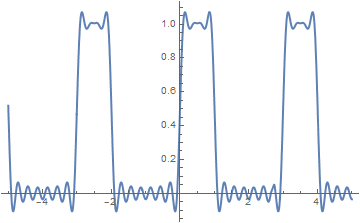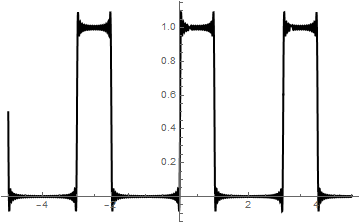We can calculate Fourier coefficients directly:
a0 = 2/3;
an = (2/3)* Integrate[Cos[n*x*2*Pi/3], {x, 0, 1}, Assumptions -> Element[n, Integers]];
bn = (2/3)* Integrate[Sin[n*x*2*Pi/3], {x, 0, 1}, Assumptions -> Element[n, Integers]];
Print[{a0, an, bn}]
fourier[m_] := a0/2 + Sum[an Cos[n x*2*Pi/3] + bn Sin[n x*2*Pi/3], {n, 1, m}]
Plot[fourier, {x, -Pi, 2*Pi}, Epilog -> {Red, PointSize[Large], Point[{{0, 0.5}, {1, 0.5}, {3, 0.5}, {-2, 0.5}, {4, 0.5}}]}, PlotStyle -> Thick]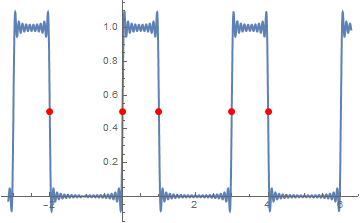Then we check numerical values of the partial sum with 30 terms at the points of discontinuity:
N[fourier /. x -> 1]
Out= 0.496939
which is closed to the true value $$\Pi (1,1,3)=1/2.$$    ■

Example 4: Consider the piecewise continuous function on the interval [-1,2]:

$f(x) = \begin{cases} 1, & \ \mbox {if } -2 < x < -1 , \\ x^2 -1, & \ \mbox {if } -1< x< 2 , \end{cases}$
Its Fourier coefficients are evaluated with the aid of Mathematica:
f[x_] = Piecewise[{{1, -2 < x < -1}, {x^2 - 1, -1 < x < 2}}, 0]
Integrate[f[x], {x, -2, 2}]/4
1/4
Other coefficients we find by direct integration:
Simplify[Integrate[f[x]*Exp[-n*Pi*I*x/2], {x, -2, 2}]/4 , Assumptions -> {Element[n, Integers], Element[x, Reals]}]
((-1)^n (8 I (-1 + I^(3 n)) + 4 (2 + I^(3 n)) n $Pi] + I (2 + I^(3 n)) n^2 \[Pi]^2))/(2 n^3 \[Pi]^3) Now we build partial sums with N = 10, 20, and 100 terms F10[x_] = 1/4 + Simplify[Sum[ (((-1)^n (8 I (-1 + I^(3 n)) + 4 (2 + I^(3 n)) n \[Pi] + I (2 + I^(3 n)) n^2 \[Pi]^2))/(2 n^3 \[Pi]^3))*Exp[n*Pi*I*x/2], {n, 1, 10}] + Sum[ (((-1)^n (8 I (-1 + I^(3 n)) + 4 (2 + I^(3 n)) n \[Pi] + I (2 + I^(3 n)) n^2 \[Pi]^2))/(2 n^3 \[Pi]^3))*Exp[n*Pi*I*x/2], {n, -10, -1}]] F20[x_] = 1/4 + Simplify[Sum[ (((-1)^n (8 I (-1 + I^(3 n)) + 4 (2 + I^(3 n)) n \[Pi] + I (2 + I^(3 n)) n^2 \[Pi]^2))/(2 n^3 \[Pi]^3))*Exp[n*Pi*I*x/2], {n, 1, 20}] + Sum[ (((-1)^n (8 I (-1 + I^(3 n)) + 4 (2 + I^(3 n)) n \[Pi] + I (2 + I^(3 n)) n^2 \[Pi]^2))/(2 n^3 \[Pi]^3))*Exp[n*Pi*I*x/2], {n, -20, -1}]] F100[x_] = 1/4 + Simplify[Sum[ (((-1)^n (8 I (-1 + I^(3 n)) + 4 (2 + I^(3 n)) n \[Pi] + I (2 + I^(3 n)) n^2 \[Pi]^2))/(2 n^3 \[Pi]^3))*Exp[n*Pi*I*x/2], {n, 1, 100}] + Sum[ (((-1)^n (8 I (-1 + I^(3 n)) + 4 (2 + I^(3 n)) n \[Pi] + I (2 + I^(3 n)) n^2 \[Pi]^2))/(2 n^3 \[Pi]^3))*Exp[n*Pi*I*x/2], {n, -100, -1}]] and plot them Plot[{f[x], F10[x]}, {x, -2.5, 2.5}, PlotStyle -> {{Thick, Black}, {Thick, Red}}] Plot[{f[x], F20[x]}, {x, -2.5, 2.5}, PlotStyle -> {{Thick, Black}, {Thick, Orange}}] Plot[{f[x], F100[x]}, {x, -2.5, 2.5}, PlotStyle -> {{Thick, Black}, {Thick, Blue}}] Fourier approximation with 10 terms Fourier approximation with 20 terms Fourier approximation with 100 terms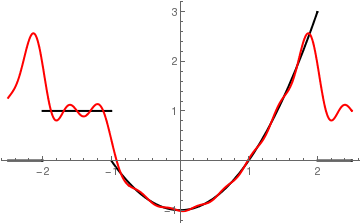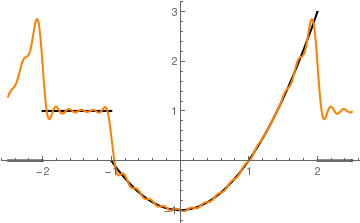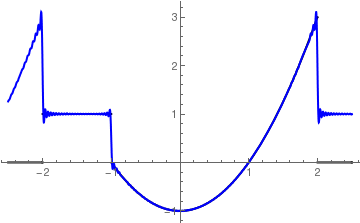■ Example 5: Let us consider the Heaviside function on a symmetric interval \[ H(x) = \begin{cases} 1, & \ \mbox {if } 0 < x < \ell , \\ 0, & \ \mbox {if } -\ell < x< 0 . \end{cases}$
Expanding the Heaviside function into the Fourier series
$H(x) = \mbox{P.V.} \sum_{k=-\infty}^{\infty} \alpha_k e^{k{\bf j} \pi x/\ell} = \lim_{N\to\infty} \sum_{k=-N}^{N} \alpha_k e^{k{\bf j} \pi x/\ell} ,$
where
$\alpha_k = \frac{1}{2\ell} \int_{0}^{\ell} e^{-k{\bf j} \pi x/\ell} \,{\text d} x = \frac{\bf j}{2k\pi} \left( 1 - e^{{\bf j}k\pi} \right)$
Integrate[Exp[k*I*Pi*x/L], {x, 0, L}]/2/L
-((I (-1 + E^(I k $Pi])))/(2 k \[Pi])) Since $$e^{{\bf j}k\pi} = (-1)^k ,$$ , we get \[ H(x) = \frac{1}{2} + \frac{2}{\pi} \sum_{k\ge 0} \frac{1}{2k+1}\,\sin \frac{\left( 2k+1 \right) \pi x}{\ell} .$
FourierSeries[HeavisideTheta[t], t, 3]
1/2 + (I E^(-I t))/$Pi] - (I E^(I t))/\[Pi] + (I E^(-3 I t))/( 3 \[Pi]) - (I E^(3 I t))/(3 \[Pi]) We check the series by plotting partial sums. S20[x_] = 1/2 + (2/Pi)*Sum[Sin[(2*k + 1)*Pi*x]/(2*k + 1), {k, 0, 20}]; Plot[S20[x], {x, -2, 2}, PlotStyle -> Thick] However, if you integrate Eq.\eqref{EqComplex.4} term-by-term, you will get another Fourier series expansion \[ h(x) = \frac{1}{2} + \frac{x\pi}{2\ell} + \frac{1}{\pi} \sum_{k\ge 1} \frac{1}{k}\,\sin \left( \frac{k\pi x}{\ell} \right) .$
Actually, the function h(x) is a ladder function that coinside with the Heaviside function on the interval (−2ℓ, 2ℓ), as the folloing plots confirm:
SS20[x_] = 1/2 + (x/2) + (1/Pi)*Sum[Sin[k*Pi*x]/(k), {k, 1, 20}];
Plot[S20[x], {x, -3, 5}, PlotStyle -> Thick]
Plot[SS20[x], {x, -3, 5}, PlotStyle -> Thick]
   Fourier approximation with 20 terms of H(x)    Fourier approximation with 20 terms of h(x)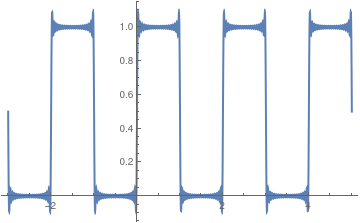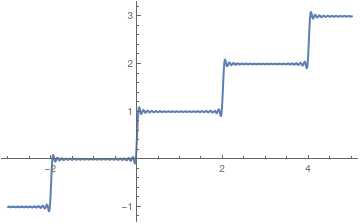■